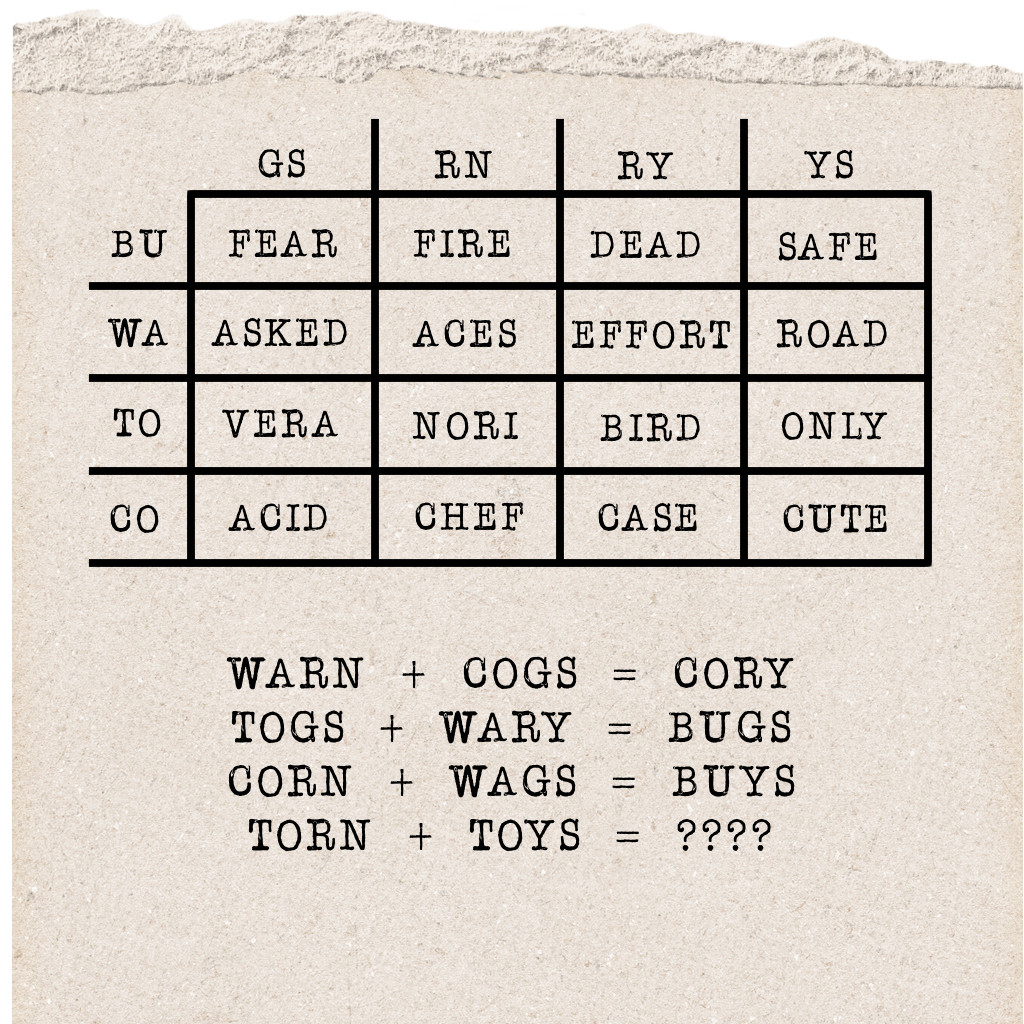Can you find the secret word?

Once you’ve solved the puzzle, use the answer as the password below (all lowercase).

The words in the equations are directing you to the correct words to look in the table. Find the box where the first two and last two letters of the words intersect in order to find the words that actually belong in the equation.

Example: WA + RN intersect at the word “ACES,” so you’ll want to use “ACES” as the first word in the first equation.

The full equations are:

ACES + ACID = CASE

VERA + EFFORT = FEAR

NORI + ONLY = ????

Now that you have the full word equations, the next step is to find the pattern they each share. Once you do, use the pattern to figure out the secret word.

Note 1: The secret word is NOT in the table.

A final hint: The 1st letter of each answer word is the 2nd letter in the 2nd word of each equation.